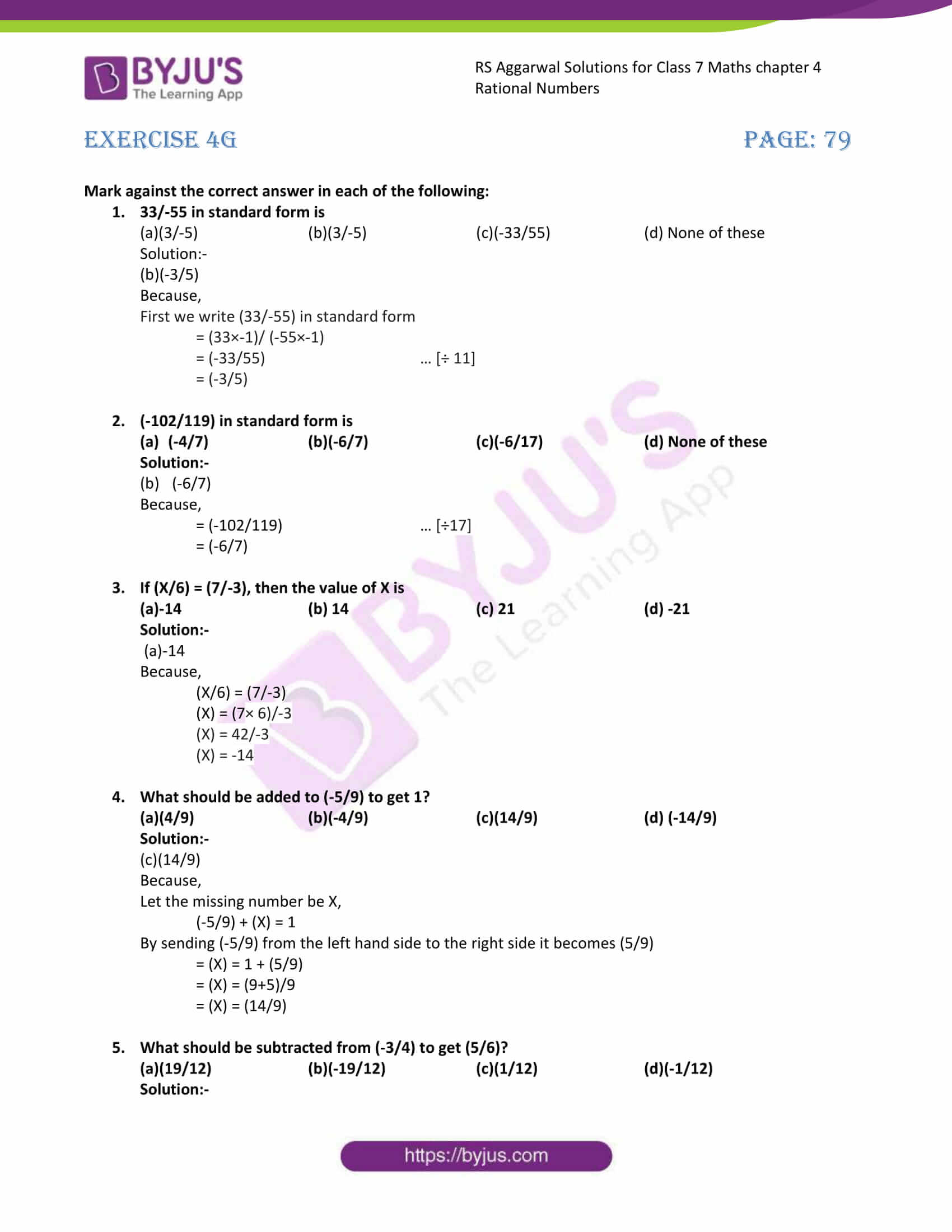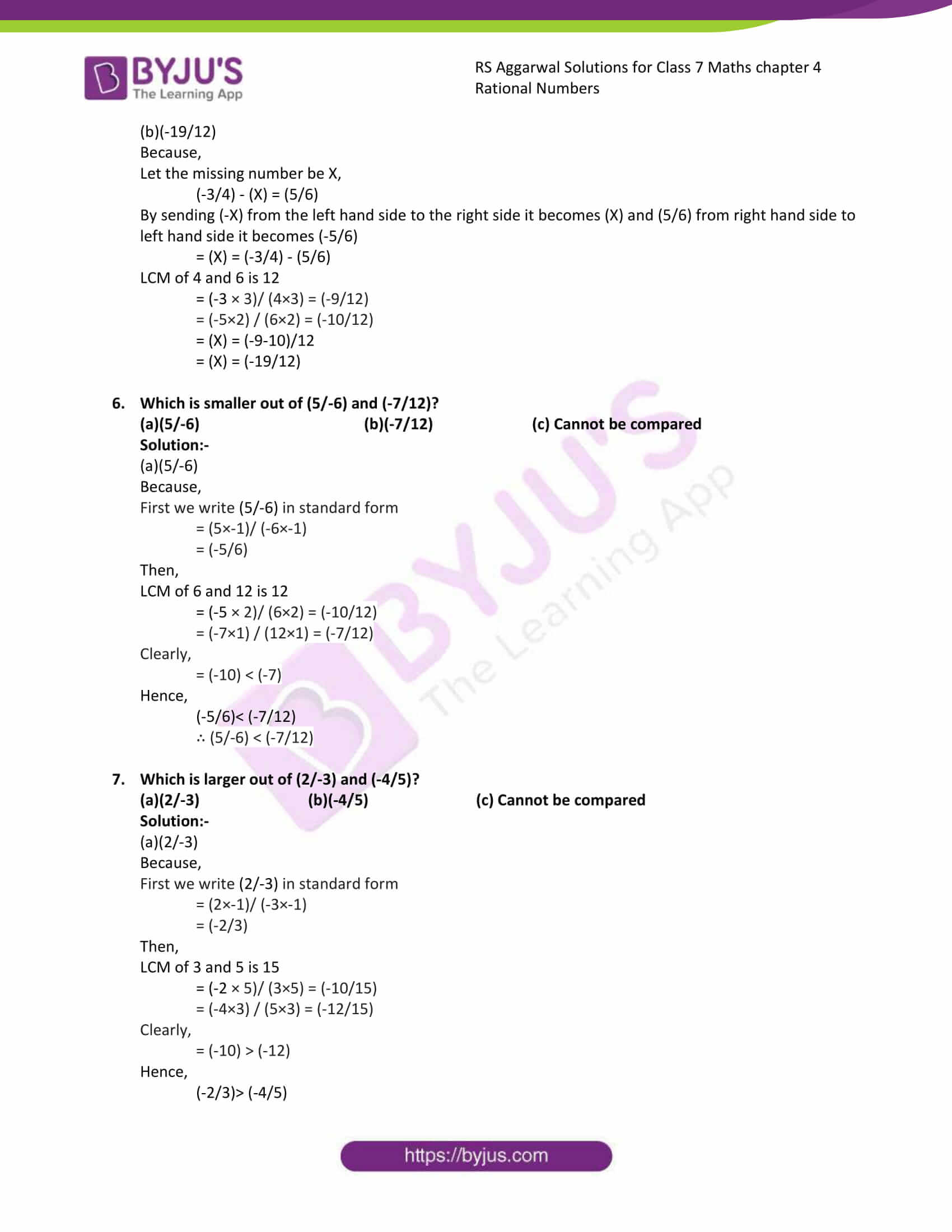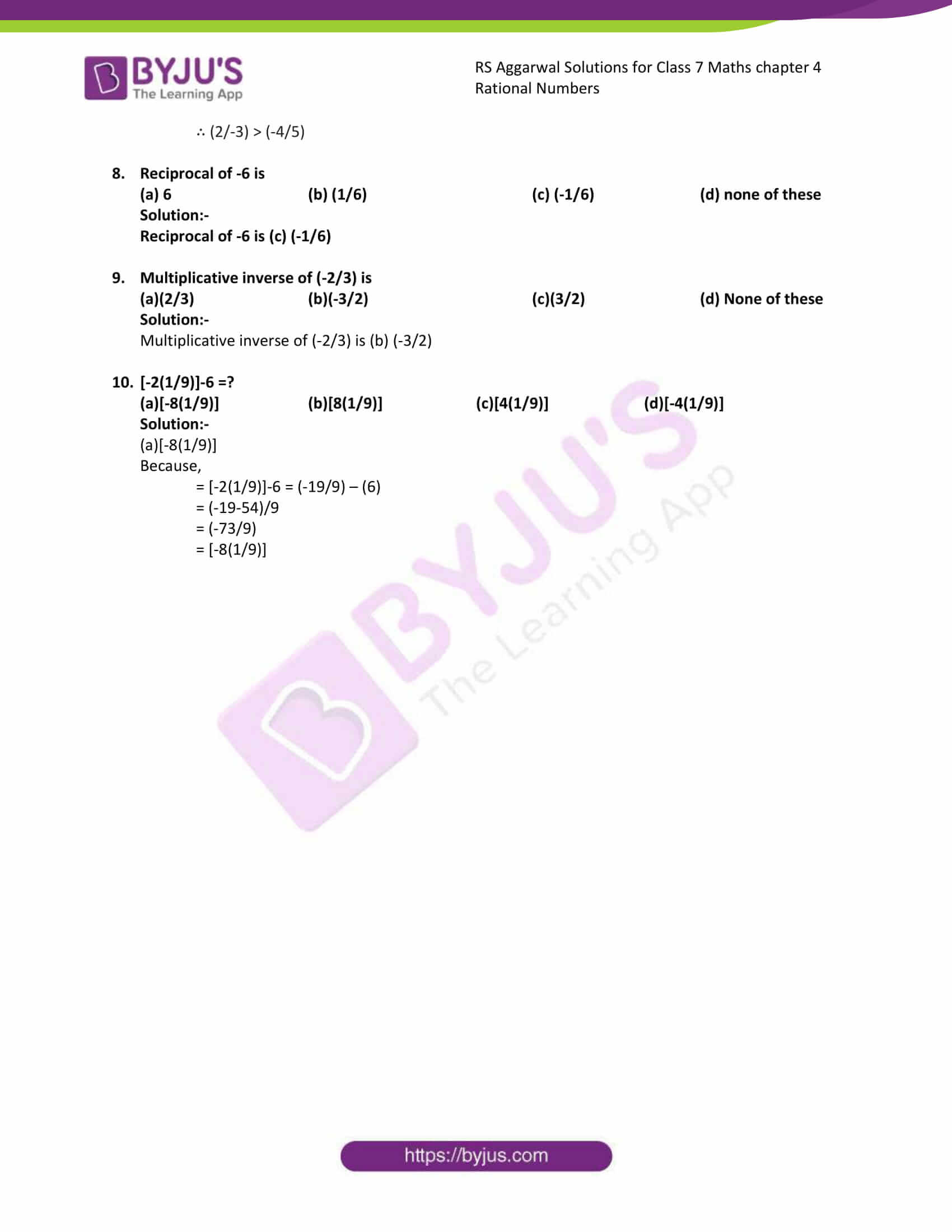# RS Aggarwal Solutions for Class 7 Maths Exercise 4G Chapter 4 Rational Numbers

RS Aggarwal Solutions for Class 7 Maths Exercise 4G Chapter 4 Rational Numbers in simple PDF are available here. This exercise is of entirely objective type questions. By solving objective type questions students will be able to solve lengthy questions in a shorter duration of time. It covers all topics present in the RS Aggarwal Solutions for Class 7 Maths Chapter 4 Rational Numbers. This would lead to them scoring good marks in Maths.

## Download the PDF of RS Aggarwal Solutions For Class 7 Maths Chapter 4 Rational Numbers – Exercise 4G### Access answers to Maths RS Aggarwal Solutions for Class 7 Chapter 4 – Rational Numbers Exercise 4G

Mark against the correct answer in each of the following:

1. 33/-55 in standard form is

(a)(3/-5) (b)(3/-5) (c)(-33/55) (d) None of these

Solution:-

(b)(-3/5)

Because,

First we write (33/-55) in standard form

= (33×-1)/ (-55×-1)

= (-33/55) … [÷ 11]

= (-3/5)

2. (-102/119) in standard form is
(-4/7) (b)(-6/7) (c)(-6/17) (d) None of these

Solution:-

(-6/7)

Because,

= (-102/119) … [÷17]

= (-6/7)

3. If (X/6) = (7/-3), then the value of X is

(a)-14 (b) 14 (c) 21 (d) -21

Solution:-

(a)-14

Because,

(X/6) = (7/-3)

(X) = (7× 6)/-3

(X) = 42/-3

(X) = -14

4. What should be added to (-5/9) to get 1?

(a)(4/9) (b)(-4/9) (c)(14/9) (d) (-14/9)

Solution:-

(c)(14/9)

Because,

Let the missing number be X,

(-5/9) + (X) = 1

By sending (-5/9) from the left hand side to the right side it becomes (5/9)

= (X) = 1 + (5/9)

= (X) = (9+5)/9

= (X) = (14/9)

5. What should be subtracted from (-3/4) to get (5/6)?

(a)(19/12) (b)(-19/12) (c)(1/12) (d)(-1/12)

Solution:-

(b)(-19/12)

Because,

Let the missing number be X,

(-3/4) – (X) = (5/6)

By sending (-X) from the left hand side to the right side it becomes (X) and (5/6) from right hand side to left hand side it becomes (-5/6)

= (X) = (-3/4) – (5/6)

LCM of 4 and 6 is 12

= (-3 × 3)/ (4×3) = (-9/12)

= (-5×2) / (6×2) = (-10/12)

= (X) = (-9-10)/12

= (X) = (-19/12)

6. Which is smaller out of (5/-6) and (-7/12)?

(a)(5/-6) (b)(-7/12) (c) Cannot be compared

Solution:-

(a)(5/-6)

Because,

First we write (5/-6) in standard form

= (5×-1)/ (-6×-1)

= (-5/6)

Then,

LCM of 6 and 12 is 12

= (-5 × 2)/ (6×2) = (-10/12)

= (-7×1) / (12×1) = (-7/12)

Clearly,

= (-10) < (-7)

Hence,

(-5/6)< (-7/12)

∴ (5/-6) < (-7/12)

7. Which is larger out of (2/-3) and (-4/5)?

(a)(2/-3) (b)(-4/5) (c) Cannot be compared

Solution:-

(a)(2/-3)

Because,

First we write (2/-3) in standard form

= (2×-1)/ (-3×-1)

= (-2/3)

Then,

LCM of 3 and 5 is 15

= (-2 × 5)/ (3×5) = (-10/15)

= (-4×3) / (5×3) = (-12/15)

Clearly,

= (-10) > (-12)

Hence,

(-2/3)> (-4/5)

∴ (2/-3) > (-4/5)

8. Reciprocal of -6 is

(a) 6 (b) (1/6) (c) (-1/6) (d) none of these

Solution:-

Reciprocal of -6 is (c) (-1/6)

9. Multiplicative inverse of (-2/3) is

(a)(2/3) (b)(-3/2) (c)(3/2) (d) None of these

Solution:-

Multiplicative inverse of (-2/3) is (b) (-3/2)

10. [-2(1/9)]-6 =?

(a)[-8(1/9)] (b)[8(1/9)] (c)[4(1/9)] (d)[-4(1/9)]

Solution:-

(a)[-8(1/9)]

Because,

= [-2(1/9)]-6 = (-19/9) – (6)

= (-19-54)/9

= (-73/9)

= [-8(1/9)]

### Access other exercises of RS Aggarwal Solutions For Class 7 Chapter 4 – Rational Numbers

Exercise 4F Solutions